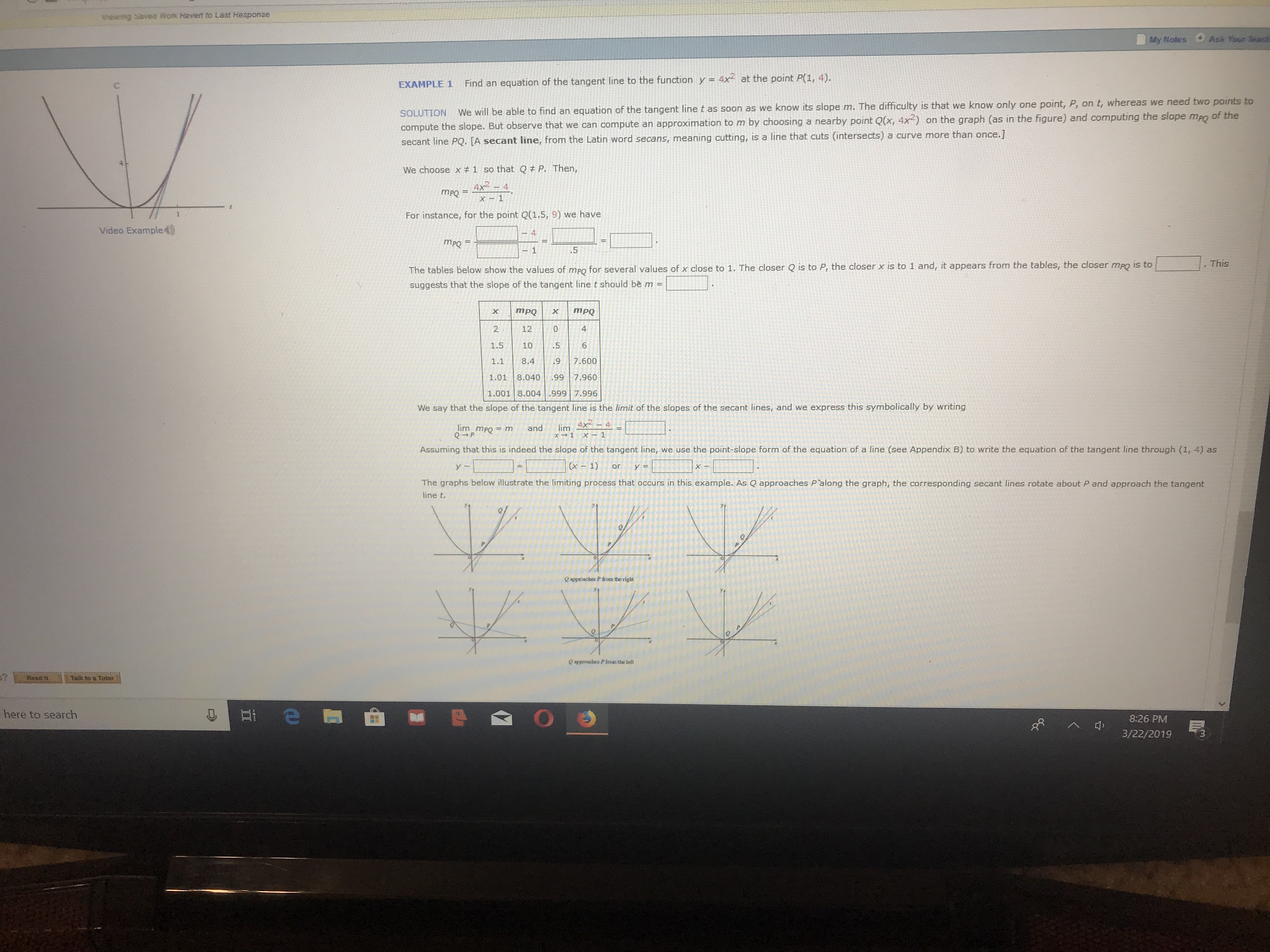# hewng Savea work Revert to Last HesponseMy Notes Ask Your leacFind an equation of the tangert line to the function y4x2 at the point P(1,4).EXAMPLE 1now only one point, P, on t, whereas we need two points toSOLUTION We will be able to find an equation of the tangent line t as soon as we know its slope m. The difficulty is that we kcompute the slope. But observe that we can compute an approximation to m by choosing a nearby point Qx, 42) on the graph (as in the figure) and computing the slope mag of theA secant line, from the Latin word secans, meaning cutting, is a line that cuts (intersects) a curve more than once.]We choose x# 1 so that Q P. Then,mpQFor instance, for the point Q(1.5,9) we haveVideo Example. ThisThe tables below show the values of mpo for several values of x close to 1. The closer Q is to P, the closer x is to 1 and, it appears from the tables, the closer meo is tosuggests that the slope of the tangent line t should be m21241.5 10.56001.01 8.040 99 7.960.001 8.004 .999 7.9968.4We say that the slope of the tangent line is the limit of the slopes of the secant lines, and we express this symbolically by writinglimmPO=mandlim 4x--4Assuming that this is indeed the slope of the tangent line, we use the point-slope form of the equation of a line (see Appendix B) to write the equation of the tangent line through (1, 4) as(x 1)The graphs below illustrate the limiting process that occurs in this example. As Q approaches Palong the graph, the corresponding secant lines rotate about P and approach the tangentline tQ appeoaches P lrom the righeTalk to Tutorhere to search8:26 PMM3/22/20193

Question
200 viewshelp_outlineImage Transcriptionclosehewng Savea work Revert to Last Hesponse My Notes Ask Your leac Find an equation of the tangert line to the function y 4x2 at the point P(1,4). EXAMPLE 1 now only one point, P, on t, whereas we need two points to SOLUTION We will be able to find an equation of the tangent line t as soon as we know its slope m. The difficulty is that we k compute the slope. But observe that we can compute an approximation to m by choosing a nearby point Qx, 4 2) on the graph (as in the figure) and computing the slope mag of the A secant line, from the Latin word secans, meaning cutting, is a line that cuts (intersects) a curve more than once.] We choose x# 1 so that Q P. Then, mpQ For instance, for the point Q(1.5,9) we have Video Example . This The t ables below show the values of mpo for several values of x close to 1. The closer Q is to P, the closer x is to 1 and, it appears from the tables, the closer meo is to suggests that the slope of the tangent line t should be m 2 12 4 1.5 10.5 600 1.01 8.040 99 7.960 .001 8.004 .999 7.996 8.4 We say that the slope of the tangent line is the limit of the slopes of the secant lines, and we express this symbolically by writing limmPO=m and lim 4x--4 Assuming that this is indeed the slope of the tangent line, we use the point-slope form of the equation of a line (see Appendix B) to write the equation of the tangent line through (1, 4) as (x 1) The graphs below illustrate the limiting process that occurs in this example. As Q approaches Palong the graph, the corresponding secant lines rotate about P and approach the tangent line t Q appeoaches P lrom the righe Talk to Tutor here to search 8:26 PMM 3/22/2019 3 fullscreen
check_circle

Step 1

To find an equation of the tangent line to the fnction y = 4x2 at the point P(1,4).

Compute an approximation to m by choosing a nearby point Q(x,4x2 ) on the graph .

Choose x≠1 so that Q≠P. Find the value of m for the point Q(1.5, 9).

Step 2

The table shows the values of m for several values of x close to 1. The closer P is to Q, the closer x is to 1.

The closer mPQ is to 8.

Step 3

The slope of the tangent line is the limit...

### Want to see the full answer?

See Solution

#### Want to see this answer and more?

Solutions are written by subject experts who are available 24/7. Questions are typically answered within 1 hour.*

See Solution
*Response times may vary by subject and question.
Tagged in

### Limits